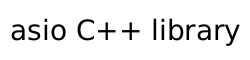##### basic_signal_set::basic_signal_set (7 of 8 overloads)

Construct a signal set and add three signals.

```basic_signal_set(
const executor_type & ex,
int signal_number_1,
int signal_number_2,
int signal_number_3);
```

This constructor creates a signal set and registers for three signals.

###### Parameters

ex

The I/O executor that the signal set will use, by default, to dispatch handlers for any asynchronous operations performed on the signal set.

signal_number_1

The first signal number to be added.

signal_number_2

The second signal number to be added.

signal_number_3

The third signal number to be added.

###### Remarks

This constructor is equivalent to performing:

```asio::signal_set signals(ex);